# Mensuration Chapter 10 Exercise 10.2 and 10.3

In this page we have NCERT Solutions for Class 6 Maths Mensuration Chapter 10 Exercise 10.2 and 10.3 . Hope you like them and do not forget to like , social share and comment at the end of the page.

## Exercise 10.2

Question 1 Find the areas of the following figures by counting square: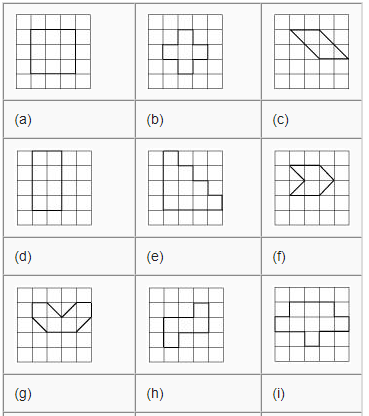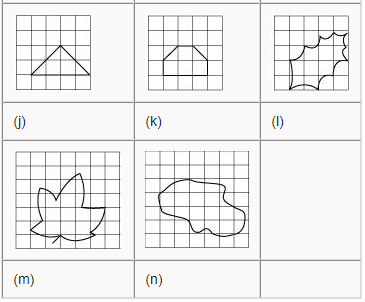Important points to consider before solving these questions
(i) The area of one full square is taken as 1 sq. unit. If it is a centimetre square sheet, then area of one full square will be 1 sq. cm.
(ii) Ignore portions of the area that are less than half a square.
(iii) If more than half of a square is in a region, just count it as one square.
(iv) If exactly half the square is counted, take its area as ½ sq. unit.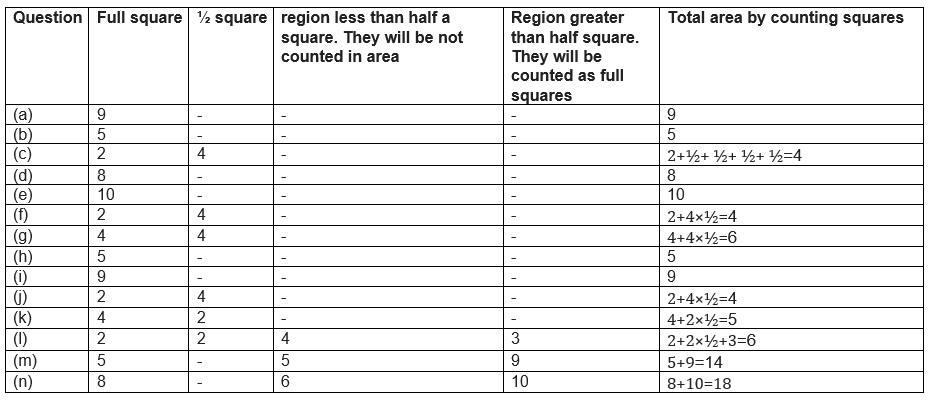## Exercise 10.3

Question 1
Find the areas of the rectangles whose sides are:
(a) 3 cm and 4 cm
(b) 12 m and 21 m
(c) 2 km and 3 km
(d) 2 m and 70 cm
Area of the rectangles is given by = $L \times B$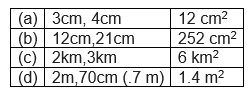Question 2
Find the areas of the squares whose sides are:
(a) 10 cm
(b) 14 cm
(c) 5 m
Area of the square is given by = $(side)^2$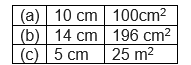Question 3
The length and breadth of three rectangles are as given below:
(a) 9 m and 6 m
(b) 17 m and 3 m
(c) 4 m and 14 m
Which one has the largest area and which one has the smallest?
Area of the rectangles is given by = $L \times B$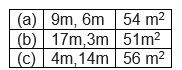(c) has the largest area and (b) has the smallest area.

Question 4
The area of a rectangular garden 50 m long is 300 sq. m. Find the width of the garden.
Length of the rectangular garden is 50 m
Area = 300 sq. m
Area of a rectangle = $length \times breadth$
or $300 = 50 \times \; breadth$
$Breadth = \frac {300}{ 50} = 6 m$
So, breadth (width) of the garden is 6 m.

Question 5
What is the cost of tiling a rectangular plot of land 500 m long and 200 m wide at the rate of Rs 8 per hundred sq. m?
To tile a rectangular plot, we need to find the area of the plot.
Given length of the plot = 500 m
Width of the plot = 200 m
So, area of the plot = $500 \times 200$ = 1,00,000 sq. m
The cost of tiling 100 sq. m = Rs 8.
So, the cost of tiling 1,00,000 sq. m is (8 × 1,00,000)/100 = Rs. 8,000

Question 6
A table-top measures 2 m by 1 m 50 cm. What is its area in square meters?
The important thing in these question is the Unit conversion. We need to either convert m into cm or cm into m. It is good to convert into lowest unit to make it easier most of the time.
Here since the answer is required in square meters, we will convert into meter only
Length of the table-top = 2 m
Width of the table-top = 1 m 50 cm = 1.50 m
So, area of the table-top(square meter) = $length \; \times \; breadth = 2 \times 1.50 = 3 \; m^2$

Question 7
A room is 4 m long and 3 m 50 cm wide. How many square meters of carpet is needed to cover the floor of the room?
This is similar to previous question. Here since the answer is required in square meters, we will convert into meter only
Length of the room = 4 m
Width of the room is 3 m 50 cm = 3.50 m
To carpet the room, we need to find the area of the floor.
So, square meters of carpet required = Area of the room = $length \; \times \; breadth = 4 \times 3.50 = 14 \; m^2$

Question 8
A floor is 5 m long and 4 m wide. A square carpet of sides 3 m is laid on the floor. Find the area of the floor that is not carpeted.
Given Length of the floor = 5 m
Width of the floor = 4 m
Total area of the floor = $lenght \; \times \; width= 5 \times 4 = 20 \; m^2$
Area of the square carpet =$lenght \; \times \; width= 3 \times; 3 = 9 \; m^2$
So, 9 sq. m of the floor is covered with carpet.
So, area of the floor that is not carpeted = $20 - 9 = 11 \; m^2$

Question 9
Five square flower beds each of sides 1 m are dug on a piece of land 5 m long and 4 m wide. What is the area of the remaining part of the land?
Area of the piece of land = $5 \times 4 = 20 \; m^2$
Area of each flower bed = $1 \times 1 = 1 \; m^2$
Five square beds are dug on the land.
So, area of five such flower beds = $5 \; m^2$
Area of the remaining part = Area of the piece of land – area of the 5 flower beds.
$= 20 - 5 = 15 \; m^2$

Question 10
By splitting the following figures into rectangles, find their areas (The measures are given in centimeters).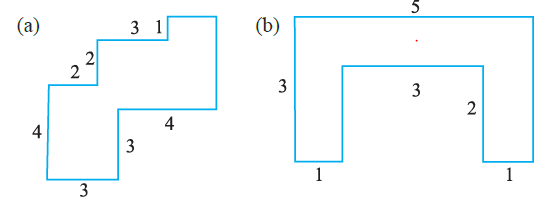(a) The given figure can be divided into four rectangles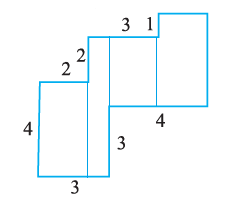Area of first rectangle = $4 \times 2=8 \; cm^2$
Area of second rectangles= $6 \times 1=6 \; cm^2$
Area of Third rectangles= $3 \times 2=6 \; cm^2$
Area of four rectangles= $4 \times 2=8 \; cm^2$
Total area =$28 \; cm^2$
(b) The given figure can be divided into three rectangles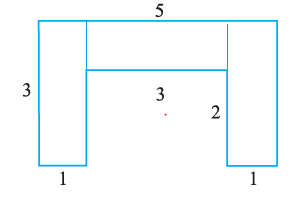Area of first rectangle = $3 \times 1=3 \; cm^2$
Area of second rectangles= $3 \times 1=3 \; cm^2$
Area of Third rectangles= $3 \times 1=3 \; cm^2$
Total area =$9 \; cm^2$

Question 11
Split the following shapes into rectangles and find their areas. (The measures are given in centimeters)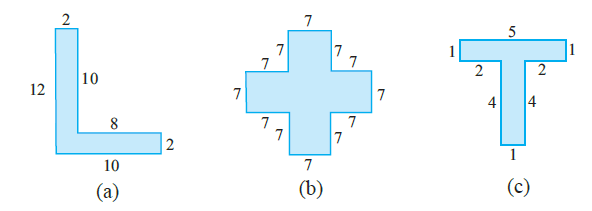This question can be attempted in the same way as previous question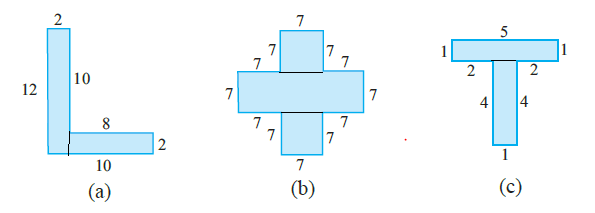(a) $40 \;cm^2$
(b) $49 \; cm^2$
(c) $9 \; cm^2$

Question 12
How many tiles whose length and breadth are 12 cm and 5 cm respectively will be needed to fit in a rectangular region whose length and breadth are respectively:
(a) 100 cm and 144 cm
(b) 70 cm and 36 cm
Length of the tile = 12 cm; Breadth of the tile = 5 cm
Area of one tile = $12 \times 5 = 60cm^2$
(a) Length of the rectangular region = 100 cm
Breadth of the rectangular region = 144 cm
Area of the rectangular region = $100 \times 144 = 14400cm^2$
So, number of tiles needed = $\frac {14400}{60} = 240 tiles$
(b) Length of the rectangular region = 70 cm
Breadth of the rectangular region = 36 cm
Area of the rectangular region = $70 \times 36 = 2520cm^2$
Therefore, number of tiles needed = $\frac {2520}{60} = 42$ tiles

A challenge!
On a centimetre squared paper, make as many rectangles as you can, such that
the area of the rectangle is 16 sq cm (consider only natural number lengths).
(a) Which rectangle has the greatest perimeter?
(b) Which rectangle has the least perimeter?
If you take a rectangle of area 24 sq cm, what will be your answers? Given any area, is it possible to predict the shape of the rectangle with the
greatest perimeter? With the least perimeter? Give example and reason
Solution
For 16sq cm, following rectangles can be drawn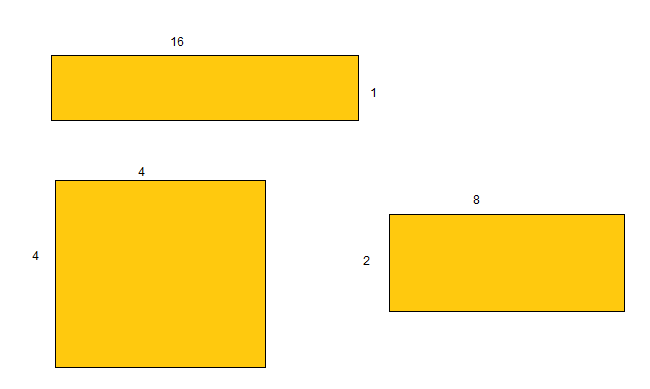Greatest Perimeter =2 (16 + 1) =34 cm
Least Perimeter = 2(4 + 4) =16 cm
For 24sq cm, following rectangles can be drawn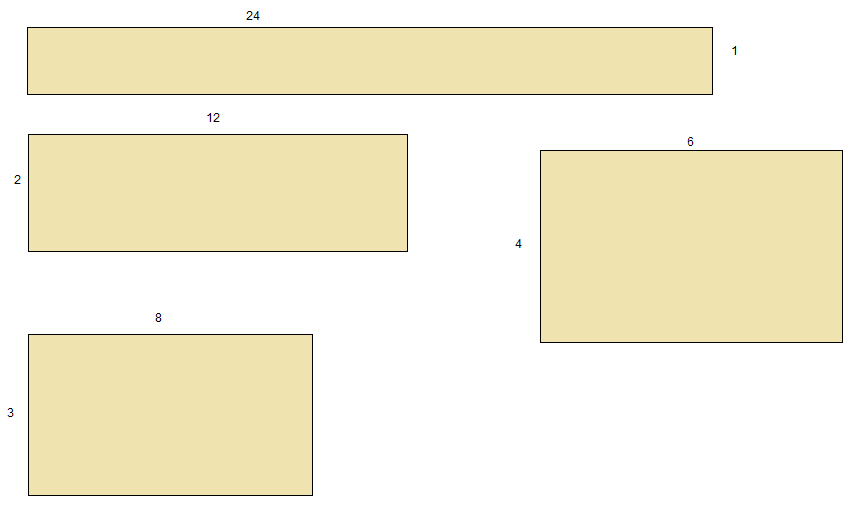Greatest Perimeter =2 (24 + 1) =50 cm
Least Perimeter = 2(6 + 4) =20 cm
Lets take one more area 18 sq cm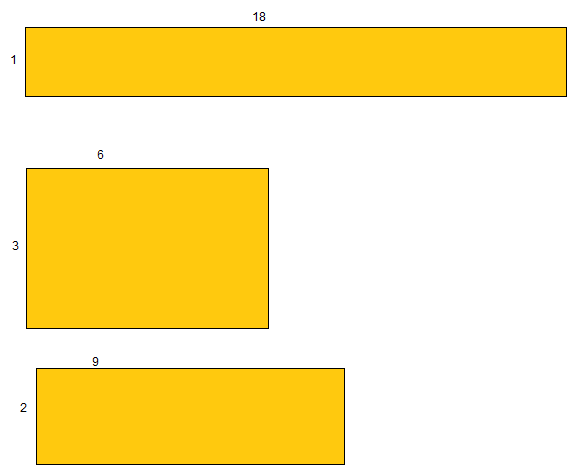Greatest Perimeter =2 (18 + 1) =38 cm
Least Perimeter = 2(6 + 3) =18 cm
Here we can a pattern for the Greatest Perimeter as
=2 (A + 1)
So given the area, we can predict the greatest perimeter
But we dont see any pattern for least perimeter. So we cannot predict### Practice Question

Question 1 What is $\frac {1}{2} + \frac {3}{4}$ ?
A)$\frac {5}{4}$
B)$\frac {1}{4}$
C)$1$
D)$\frac {4}{5}$
Question 2 Pinhole camera produces an ?
A)An erect and small image
B)an Inverted and small image
C)An inverted and enlarged image
D)None of the above